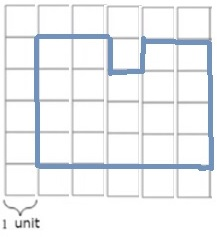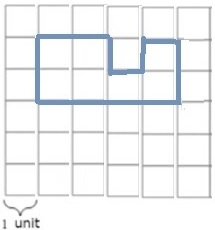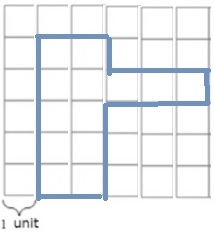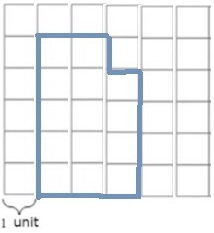# Perimeter and Area on a Grid Online Quiz

Following quiz provides Multiple Choice Questions (MCQs) related to Perimeter and Area on a Grid. You will have to read all the given answers and click over the correct answer. If you are not sure about the answer then you can check the answer using Show Answer button. You can use Next Quiz button to check new set of questions in the quiz.Q 1 - Find the perimeter of the shape on the gridA - 22 units

B - 24 units

C - 20 units

D - 23 units

### Explanation

Perimeter =

The length of the boundary of the shape on grid =

= 5 + 5 + 5 + 2 + 1 + 1 + 1 + 2 = 22 units

Q 2 - Find the perimeter of the shape on the grid.A - 22 units

B - 24 units

C - 20 units

D - 23 units

### Explanation

Perimeter =

The length of the boundary of the shape on grid

= 4 + 5 + 4 + 2 + 1 + 1 + 1 + 2 = 20 units

Q 3 - Find the perimeter of the shape on the grid.A - 22 units

B - 18 units

C - 20 units

D - 21 units

### Explanation

Perimeter =

The length of the boundary of the shape on grid

= 3 + 5 + 3 + 2 + 1 + 1 + 1 + 2 = 18 units

Q 4 - Find the perimeter of the shape on the grid.A - 22 units

B - 18 units

C - 20 units

D - 16 units

### Explanation

Perimeter =

The length of the boundary of the shape on grid

= 2 + 5 + 2 + 2 + 1 + 1 + 1 + 2 = 16 units

Q 5 - Find the perimeter of the shape on the grid.A - 14 units

B - 18 units

C - 20 units

D - 16 units

### Explanation

Perimeter =

The length of the boundary of the shape on grid =

= 2 + 4 + 2 + 2 + 1 + 1 + 1 + 1 = 14 units

Q 6 - Find the area of the shape on the grid.A - 15 square units

B - 14 square units

C - 13 square units

D - 12 square units

### Explanation

Step 1:

The total number of full unit squares occupied by the shape on grid = 13

Area of each unit square = 1 square units

Step 2:

Area of shape on grid = 13 square units

Q 7 - Find the area of the shape on the grid.A - 18 square units

B - 19 square units

C - 17 square units

D - 16 square units

### Explanation

Step 1:

The total number of full unit squares occupied by the shape on grid = 19

Area of each unit square = 1 square units

Step 2:

Area of shape on grid = 19 square units

Q 8 - Find the area of the shape on the grid.A - 15 square units

B - 14 square units

C - 13 square units

D - 12 square units

### Explanation

Step 1:

The total number of full unit squares occupied by the shape on grid = 14

Area of each unit square = 1 square units

Step 2:

Area of shape on grid = 14 square units

Q 9 - Find the area of the shape on the grid.A - 15 square units

B - 14 square units

C - 13 square units

D - 11 square units

### Explanation

Step 1:

The total number of full unit squares occupied by the shape on grid = 11

Area of each unit square = 1 square units

Step 2:

Area of shape on grid = 11 square units

Q 10 - Find the area of the shape on the grid.A - 15 square units

B - 14 square units

C - 13 square units

D - 11 square units

### Explanation

Step 1:

The total number of full unit squares occupied by the shape on grid = 13

Area of each unit square = 1 square units

Step 2:

Area of shape on grid = 13 square units

perimeter_and_area_on_grid.htm